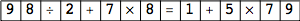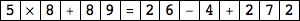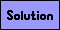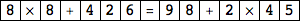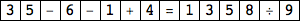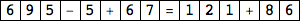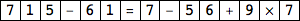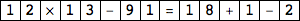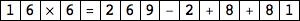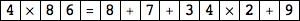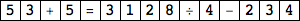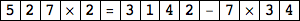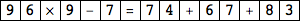Blackout Puzzles

Each of the puzzles below is actually 3 puzzles in 1. First black out 2 of the squares so that the remaining equation is true. Then black out 3 different squares to make a correct equation. Then do it with 4 squares. The standard order of operations is used: multiplication and division are done before addition and subtraction. Only whole numbers are used, though the results might be negative. A sample puzzle is shown below.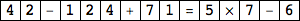→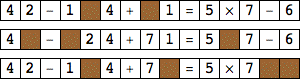Each puzzle below has a unique solution.